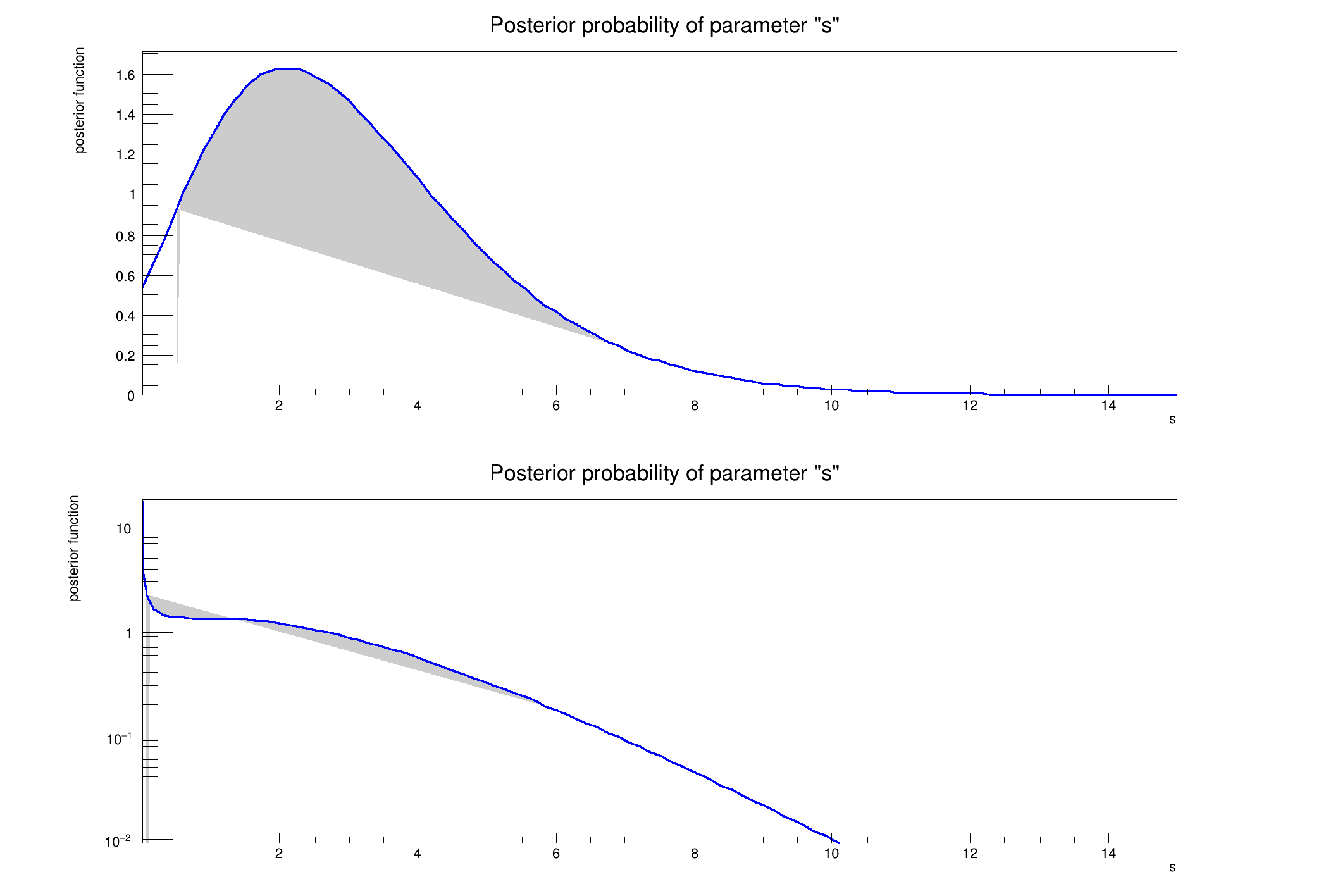ROOT   Reference Guiders701_BayesianCalculator.C File Reference

## Detailed Description␛[1mRooFit v3.60 -- Developed by Wouter Verkerke and David Kirkby␛[0m
Copyright (C) 2000-2013 NIKHEF, University of California & Stanford University
[#1] INFO:ObjectHandling -- RooWorkspace::exportToCint(w) INFO: references to all objects in this workspace will be created in CINT in 'namespace w'
Bayesian Result using a Flat prior
0.9% CL central interval: [ 0.50606 - 6.89326 ] or 0.95% CL limits
Bayesian Result using a 1/sqrt(s) prior
0.9% CL central interval: [ 0.0746294 - 5.85425 ] or 0.95% CL limits
#include "RooRealVar.h"
#include "RooWorkspace.h"
#include "RooDataSet.h"
#include "RooPlot.h"
#include "RooMsgService.h"
#include "TCanvas.h"
using namespace RooFit;
using namespace RooStats;
void rs701_BayesianCalculator(bool useBkg = true, double confLevel = 0.90)
{
RooWorkspace *w = new RooWorkspace("w", true);
w->factory("SUM::pdf(s[0.001,15]*Uniform(x[0,1]),b[1,0,2]*Uniform(x))");
w->factory("Gaussian::prior_b(b,1,1)");
w->factory("PROD::model(pdf,prior_b)");
RooAbsPdf *model = w->pdf("model"); // pdf*priorNuisance
RooArgSet nuisanceParameters(*(w->var("b")));
RooAbsRealLValue *POI = w->var("s");
RooAbsPdf *priorPOI = (RooAbsPdf *)w->factory("Uniform::priorPOI(s)");
RooAbsPdf *priorPOI2 = (RooAbsPdf *)w->factory("GenericPdf::priorPOI2('1/sqrt(@0)',s)");
w->factory("n"); // observed number of events
// create a data set with n observed events
RooDataSet data("data", "", RooArgSet(*(w->var("x")), *(w->var("n"))), "n");
// to suppress messages when pdf goes to zero
RooArgSet *nuisPar = 0;
if (useBkg)
nuisPar = &nuisanceParameters;
// if (!useBkg) ((RooRealVar *)w->var("b"))->setVal(0);
double size = 1. - confLevel;
std::cout << "\nBayesian Result using a Flat prior " << std::endl;
BayesianCalculator bcalc(data, *model, RooArgSet(*POI), *priorPOI, nuisPar);
bcalc.SetTestSize(size);
SimpleInterval *interval = bcalc.GetInterval();
double cl = bcalc.ConfidenceLevel();
std::cout << cl << "% CL central interval: [ " << interval->LowerLimit() << " - " << interval->UpperLimit()
<< " ] or " << cl + (1. - cl) / 2 << "% CL limits\n";
RooPlot *plot = bcalc.GetPosteriorPlot();
TCanvas *c1 = new TCanvas("c1", "Bayesian Calculator Result");
c1->Divide(1, 2);
c1->cd(1);
plot->Draw();
c1->Update();
std::cout << "\nBayesian Result using a 1/sqrt(s) prior " << std::endl;
BayesianCalculator bcalc2(data, *model, RooArgSet(*POI), *priorPOI2, nuisPar);
bcalc2.SetTestSize(size);
SimpleInterval *interval2 = bcalc2.GetInterval();
cl = bcalc2.ConfidenceLevel();
std::cout << cl << "% CL central interval: [ " << interval2->LowerLimit() << " - " << interval2->UpperLimit()
<< " ] or " << cl + (1. - cl) / 2 << "% CL limits\n";
RooPlot *plot2 = bcalc2.GetPosteriorPlot();
c1->cd(2);
plot2->Draw();
c1->Update();
// observe one event while expecting one background event -> the 95% CL upper limit on s is 4.10
// observe one event while expecting zero background event -> the 95% CL upper limit on s is 4.74
}
RooAbsRealLValue is the common abstract base class for objects that represent a real value that may a...
Double_t getVal(const RooArgSet *normalisationSet=nullptr) const
Evaluate object.
Definition: RooAbsReal.h:90
RooArgSet is a container object that can hold multiple RooAbsArg objects.
Definition: RooArgSet.h:28
RooDataSet is a container class to hold unbinned data.
Definition: RooDataSet.h:33
static RooMsgService & instance()
Return reference to singleton instance.
void setGlobalKillBelow(RooFit::MsgLevel level)
A RooPlot is a plot frame and a container for graphics objects within that frame.
Definition: RooPlot.h:44
The RooWorkspace is a persistable container for RooFit projects.
Definition: RooWorkspace.h:43
RooRealVar * var(const char *name) const
Retrieve real-valued variable (RooRealVar) with given name. A null pointer is returned if not found.
RooFactoryWSTool & factory()
Return instance to factory tool.
RooAbsPdf * pdf(const char *name) const
Retrieve p.d.f (RooAbsPdf) with given name. A null pointer is returned if not found.
The Canvas class.
Definition: TCanvas.h:27
return c1
Definition: legend1.C:41
The namespace RooFit contains mostly switches that change the behaviour of functions of PDFs (or othe...
Namespace for the RooStats classes.
Definition: Asimov.h:19

Definition in file rs701_BayesianCalculator.C.